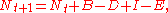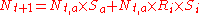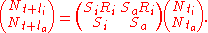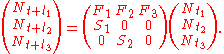xMatrix population modelsEncyclopedia
Population models are used in population ecology
Population ecology
Population ecology is a sub-field of ecology that deals with the dynamics of species populations and how these populations interact with the environment. It is the study of how the population sizes of species living together in groups change over time and space....

to model the dynamics of wildlife or human populations. Matrix population models are a specific type of population model that uses matrix algebra
Matrix algebra
Matrix algebra may refer to:*Matrix theory, is the branch of mathematics that studies matrices*Matrix ring, thought of as an algebra over a field or a commutative ring...

. Matrix algebra, in turn, is simply a form of algebraic shorthand for summarizing a larger number of often repetitious and tedious algebraic computations.

All populations can be modeled by one simple equation:where:
• Nt+1 = abundance at time t+1
• Nt = abundance at time t
• B = number of births within the population between Nt and Nt+1
• D = number of deaths within the population between Nt and Nt+1
• I = number of individuals immigrating into the population between Nt and Nt+1
• E = number of individuals emigrating from the population between Nt and Nt+1

This equation is called a BIDE model (Birth, Immigration, Death, Emigration model).

Although BIDE models are conceptually simple, reliable estimates of the 5 variables contained therein (N, B, D, I and E) are often difficult to obtain. Usually a researcher attempts to estimate current abundance, Nt, often using some form of mark and recapture
Mark and recapture
Mark and recapture is a method commonly used in ecology to estimate population size. This method is most valuable when a researcher fails to detect all individuals present within a population of interest every time that researcher visits the study area...

technique. Estimates of B might be obtained via a ratio of immatures to adults soon after the breeding season, Ri. Number of deaths can be obtained by estimating annual survival probability, usually via mark and recapture
Mark and recapture
Mark and recapture is a method commonly used in ecology to estimate population size. This method is most valuable when a researcher fails to detect all individuals present within a population of interest every time that researcher visits the study area...

methods, then multipling present abundance and survival rate
Survival rate
In biostatistics, survival rate is a part of survival analysis, indicating the percentage of people in a study or treatment group who are alive for a given period of time after diagnosis...

. Often, immigration and emigration are ignored because they are so difficult to estimate.

For added simplicity it may help to think of time t as the end of the breeding season in year t and to imagine that one is studying a species that has only one discrete breeding season per year.

The BIDE model can then be expressed as:where:
• Nt,a = number of adult females at time t
• Nt,i = number of immature females at time t
• Sa = annual survival of adult females from time t to time t+1
• Si = annual survival of immature females from time t to time t+1
• Ri = ratio of surviving young females at the end of the breeding season per breeding female

In matrix notation this model can be expressed as:Suppose that you are studying a species with a maximum lifespan of 4 years. The following is an age-based Leslie matrix for this species. Each row in the first and third matrices corresponds to animals within a given age range (0–1 years, 1–2 years and 2–3 years). In a Leslie matrix the top row of the middle matrix consists of age-specific fertilities: F1, F2 and F3. Note, that F1 = Si×Ri in the matrix above. Since this species does not live to be 4 years old the matrix does not contain an S3 term.These models can give rise to interesting cyclical or seemingly chaotic patterns in abundance over time when fertility rates are high.

The terms Fi and Si can be constants or they can be functions of environment, such as habitat or population size. Randomness can also be incorporated into the environmental component.

## Reference